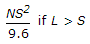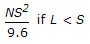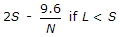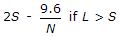# Civil Engineering - Highway Engineering - Discussion

### Discussion :: Highway Engineering - Section 1 (Q.No.20)

20.

If N is deviation angle the length L of a parabolic vetical curve for overtaking sight distance S, is

 [A].[B].[C].[D].[E]. Both (A) and (C)

Explanation:

No answer description available for this question.

 Chandu said: (Mar 22, 2018) For SSD NS^2/4.4.

 Ali Raza said: (Jan 6, 2019) The Correct answer is C only.

 Pramod said: (Jan 20, 2019) Answer E is correct. Because the formula for SSD IS g*s2*/9.6 (if s is smaller than L). If s is greater than L 2s-9. 6/g. And Non-SSD formula is g*s2/4 (if s is less than L) if s is greater than L 2s-4/g.

 Debu said: (Apr 26, 2019) Only C is correct.